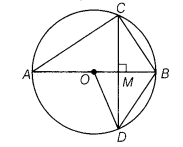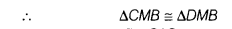# Given figure,O is the centre of the circle, BD = OD and CD intersect AB at 90°.Find ∠CAB

In the given figure, O is the centre of the circle, BD = OD and CD intersect AB at 90°. Find ∠CAB.In ∆ OBD, BD = OD
OD =OB
∴OB = OD = BD
Thus,∆ ODB is an equilateral triangle.
∴∠BOD =∠OBD =∠ODB = 60°
Now, in ∆CMB and ∆DMB, we have
MB = MB [common side]
∠CMB =∠DMB [each 90°]
CM = DM
[∵ perpendicular drawn from the centre to the chord,bisect the chord][by SAS congruence criterion]
Then, ∠MBC = ∠MBD [∵CPCT ]
∠MBC = ∠MBD = 60°
[∵ ∠OBD =∠MBD = 60°]
or ∠ABC = 60°
Since, AB is a diameter of the circle.
∴ ∠ACB = 90° [∵angle in a semi-circle is 90°]
In ∆ACS,∠CAB + ∠ABC + ∠ACB = 180°
[∵ sum of the angles of a triangle is 180°]
=>∠CAB + 60° + 90° = 180° [∵ ∠ABC= 60°]
∴ ∠CAB = 180°- (60° + 90°) = 30°